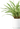# GRE Quant practice exam

Studying for the GRE Quant?

Scroll down to try 10 GRE Quantitative Reasoning practice exam questions for free.
The GRE is a standardized test for all types of graduate schools, including business and law schools. It is created and administered by Educational Testing Service (ETS).
Time
3 hours 45 minutes
Format
100 questions
2 essays
Exam fee
\$220
Details
Solve each of the GRE Quant practice test questions below to get a feel for what to expect on the actual GRE Quant exam. Achievable's free GRE Quant practice questions are scored instantly, providing the correct answer along with an easy to understand explanation. Get started on the path to passing the GRE Quant exam by solving 10 GRE Quant exam sample questions.
Question 1

The recipe demands six and three quarter cups of flour to make 21 pancakes

Quantity A
the flour required to make 22 pancakes
Quantity B
7
A.
Quantity A is greater
B.
Quantity B is greater
C.
The two quantities are equal
D.
Relationship cannot be determined
Question 2

Average price (\$) of gas by month in 2022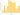Miles per gallon by vehicle brand

Vehicle brandMiles per gallon
Realm Rover31
Wolf Wagon35
Storm Sterling39
Gibraltar29
Abyss23

Based on this data, it would be most expensive to:

A.
drive 20 miles with the Gibraltar in July
B.
drive 31 miles with the Abyss in April
C.
drive 45 miles with the Storm Sterling in September
D.
drive 47 miles with the Abyss in May
E.
drive 47 miles with the Realm Rover in October
Question 3

The centerline evenly splits the triangle below with A = 7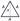Quantity A
𝑥
Quantity B
6
A.
Quantity A is greater
B.
Quantity B is greater
C.
The two quantities are equal
D.
Relationship cannot be determined
Question 4

Lines AB and CD both run through the midpoint of both the interior and exterior circles, and the two lines are perpendicular to each other. What is the total area of the shaded regions if the straight line CB (not shown) has a length equal to 8√2 and if the radius of the interior circle is exactly half the radius of the exterior circle?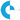A.
16π
B.
24π
C.
28π
D.
48π
E.
64π
Question 5

Given points A (-2, -2) and B (2, 7):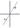Quantity A
The slope of the line that passes through points A and B
Quantity B
The distance between points A and B
A.
Quantity A is greater
B.
Quantity B is greater
C.
The two quantities are equal
D.
Relationship cannot be determined
Question 6

A group of friends agreed to buy a number of lottery tickets with each individual contributing \$8.75 to the group fund. If 2 of the friends revealed to the group that they could only contribute \$6.75 instead of the agreed \$8.75, how many people are in this friend group if the new average contribution would then be \$8.50?
A.
14
B.
15
C.
16
D.
17
E.
18
Question 7

The national test scores for a college entrance exam had a normal distribution. A total of 34,000 people took the exam, the mean score was 78, and 23,142 people scored between 74 and 82 on the exam.

Quantity A
One standard deviation
Quantity B
4
A.
Quantity A is greater
B.
Quantity B is greater
C.
The two quantities are equal
D.
Relationship cannot be determined
Question 8

There are a total of 50 students sitting in the lecture hall listening to the professor. 20% of the students in the audience are auditing the course. 30% of the auditing students are taking notes, while 70% of the entire lecture hall is not taking notes. What percent of the graded (non-audit) students are not taking notes? Round to the nearest percent.
A.
30%
B.
64%
C.
70%
D.
77%
E.
80%
Question 9

How many combinations of attire exist in my wardrobe if I have 10 pants, 2 shirts, and 10 hats. Each combination requires one of each of these clothing types.
A.
120
B.
160
C.
180
D.
200
E.
900
Question 10

Account A has an initial principal of \$9,300 with a simple interest rate of 4%. Account B has an initial principal of \$9,400 with a compound interest rate of 10%. Account B will be approximately what percent greater than Account A after 3 years? Round to the nearest percent.
A.
8%
B.
14%
C.
17%
D.
20%
E.
21%
Working through practice GRE Quant exam questions is the number one strategy for passing the GRE Quant exam. Achievable's bank of GRE Quant practice exam questions has thousands of randomized GRE Quant test questions to help you study for the GRE Quant. Using free GRE Quant practice exams is risky since most will be low-quality or outdated, but Achievable includes 200+ quant templates hand-crafted for the GRE Quant. Get started taking GRE Quant practice tests today by enrolling in Achievable's GRE course.
Achievable GRE - \$199
Hit your GRE target score on the first try with Achievable's online course. Includes everything you need: unlimited quantitative practice questions, an easy-to-read online textbook, 10 verbal / reading comprehension practice exams, 250 vocabulary words, and unlimited instant essay grading.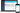ETS's GRE exam summary
Topic
Description
Quantitative Reasoning
Two sections, 20 questions each

The Quantitative Reasoning section measures your ability to:

• understand, interpret and analyze quantitative information
• solve problems using mathematical models
• apply basic skills and elementary concepts of arithmetic, algebra, geometry and data analysis

Verbal Reasoning
Two sections, 20 questions each

The Verbal Reasoning section measures your ability to:

• analyze and draw conclusions from discourse; reason from incomplete data; identify author's assumptions and/or perspective; understand multiple levels of meaning, such as literal, figurative and author's intent
• select important points; distinguish major from minor or irrelevant points; summarize text; understand the structure of a text
• understand the meanings of words, sentences and entire texts; understand relationships among words and among concepts

Analytical Writing
Two essay prompts

The Analytical Writing section measures your ability to:

• articulate complex ideas clearly and effectively
• support ideas with relevant reasons and examples
• examine claims and accompanying evidence
• sustain a well-focused, coherent discussion
• control the elements of standard written English